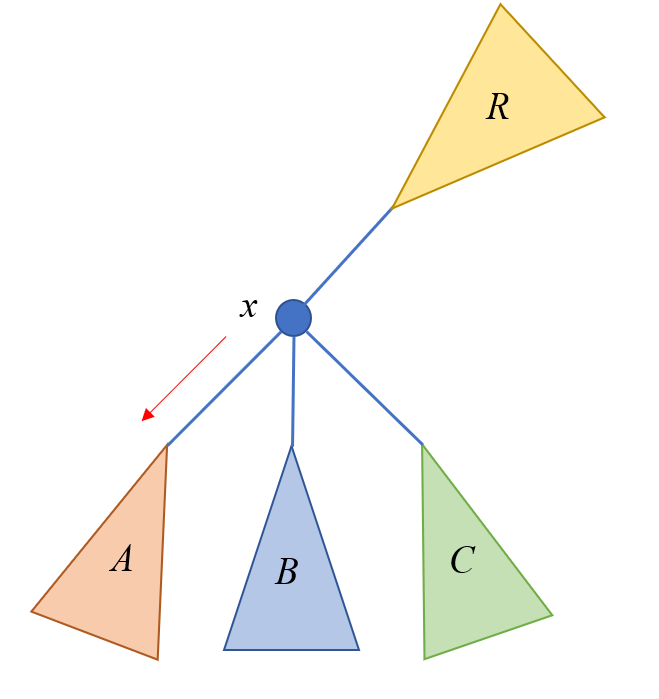# 51nod 1812 树的双直径 题解【树形DP】【贪心】

## 输入输出样例

5
1 2 8
2 3 -4
3 4 9
2 5 9


153


## 说明

$2\le n\le 4\times 10^5,|c_i|\le 10^9$

51nod 支持 int128

__int128 n;


## 题解：

trick：对于乘积最大可能产生贡献的分别是最大的两个值和最小的两个值的乘积。## Code：

#include<cstdio>
#include<cstring>
#include<algorithm>
#define ll long long
#define lll __int128
#define maintain() sort(s[x],s[x]+4,cmp),sort(s_[x],s_[x]+4),sort(t[x],t[x]+3,cmp),sort(t_[x],t_[x]+3);
using std::sort;
bool cmp(ll x,ll y){return x>y;}
ll Max(ll x,ll y){return x>y?x:y;}
ll Min(ll x,ll y){return x<y?x:y;}
struct edge
{
int n,nxt,v;
edge(int n,int nxt,int v)
{
this->n=n;
this->nxt=nxt;
this->v=v;
}
edge(){}
}e;
{
}
ll f,g;
ll f_,g_;
//第一次 dfs 求出 f,g
//f[x] 表示 x 子树中伸出来的最大值
//g[x] 表示 x 树内的最大值
//_ 表示最小值
void Dfs(int x,int from)
{
if(e[i].n!=from)
{
Dfs(e[i].n,x);
ll p=f[e[i].n]+e[i].v,p_=f_[e[i].n]+e[i].v;
g[x]=Max(Max(g[x],f[x]+p),g[e[i].n]);
g_[x]=Min(Min(g_[x],f_[x]+p_),g_[e[i].n]);
f[x]=Max(f[x],p);
f_[x]=Min(f_[x],p_);
}
g[x]=g[x]>f[x]?g[x]:f[x];
g_[x]=g_[x]<f_[x]?g_[x]:f_[x];
}
lll ans=0;
ll s,s_;//最大两个 f
ll t,t_;//最大两个 g
ll Gg,Gg_;//维护子树中最大的 g
ll F,G,F_,G_;//相当于 f[fa[x]]
void dfs(int x,int from,int h)//h 是来边
{
ans=ans>(lll)G*g[x]?ans:(lll)G*g[x];
ans=ans>(lll)G_*g_[x]?ans:(lll)G_*g_[x];
//F 更新 G
ll gg=Max(G,F);//在本层递归中存的 G
ll gg_=Min(G_,F_);

F+=h;
F_+=h;

for(int i=0;i<=3;++i)
s[x][i]=s_[x][i]=0;
for(int i=0;i<=2;++i)
t[x][i]=t_[x][i]=0;//清零

s[x]=F;//更新父亲方向的信息
s_[x]=F_;
t[x]=G;
t_[x]=G_;
maintain();

ll ff=F,ff_=F_;

if(e[i].n!=from)
{
s[x]=f[e[i].n]+e[i].v;
s_[x]=f_[e[i].n]+e[i].v;
t[x]=g[e[i].n];
t_[x]=g_[e[i].n];
maintain();
}
if(e[i].n!=from)
{
if(g[e[i].n]==t[x])//更新子树中 g 的信息
Gg=t[x];
else
Gg=t[x];

if(g_[e[i].n]==t_[x])
Gg_=t_[x];
else
Gg_=t_[x];

if(f[e[i].n]+e[i].v==s[x])//需要排除即将进去的子树
{
G=Max(Max(gg,s[x]+s[x]),Gg);
F=Max(ff,s[x]);
}
else if(f[e[i].n]+e[i].v==s[x])
{
G=Max(Max(gg,s[x]+s[x]),Gg);
F=Max(ff,s[x]);
}
else
{
G=Max(Max(gg,s[x]+s[x]),Gg);
F=Max(ff,s[x]);
}

if(f_[e[i].n]+e[i].v==s_[x])//还要更新F
{
G_=Min(Min(gg_,s_[x]+s_[x]),Gg_);
F_=Min(ff_,s_[x]);
}
else if(f_[e[i].n]+e[i].v==s_[x])
{
G_=Min(Min(gg_,s_[x]+s_[x]),Gg_);
F_=Min(ff_,s_[x]);
}
else
{
G_=Min(Min(gg_,s_[x]+s_[x]),Gg_);
F_=Min(ff_,s_[x]);
}

dfs(e[i].n,x,e[i].v);
}
}
int main()
{
int n,u,v,w;
scanf("%d",&n);
for(int i=1;i<n;++i)
{
scanf("%d%d%d",&u,&v,&w);
}
Dfs(n>>1,0);
dfs(n>>1,0,0);
if(!ans)//注意用栈输出时为 0 的情况
puts("0");
int stk,tp=0;
while(ans)
{
stk[++tp]=ans%10;
ans/=10;
}
while(tp)
printf("%d",stk[tp--]);
return 0;
}


### 3 说点什么0 Followers

Most reacted comment
2 Comment authorsRecent comment authors
Subscribeorz 考场爆零选手路过（顺便滋瓷数字汉字之间加空格的习惯qwq）wpDiscuz